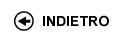Politecnico di Torino
01LQMDR, 01LQMAX, 01LQMJA
Linear algebra
Corso di Laurea in Ingegneria Meccanica - Vercelli
Corso di Laurea in Ingegneria Civile - Vercelli
Corso di Laurea in Electronic And Computer Engineering - Vercelli
 Docente Qualifica Settore Lez Es Lab Tut Anni incarico Spreafico Maria LuisaRC MAT/04 30 20 0 20 3
 SSD CFU Attivita' formative Ambiti disciplinari MAT/02 5 C - Affini o integrative Cultura scientifica, umanistica, giuridica, economica, socio-politica
Esclusioni:
01AAW
 Obiettivi dell'insegnamento The goal of this course is to learn the fundamental tools of linear algebra which are basic for engineering applications. Competenze attese At the end of the course the student will be able to use matrix calculus, to solve linear systems, to compute dimension, bases, intersections and unions of vector and subvector spaces, to compute eigenvalues, eigenvectors with applications to diagonalization of square matrices and to the study of conics in the plane. Prerequisiti The students are expected to know results and techniques from trigonometry, complex numbers and basic mathematic of hight school. Programma - Matrices (sum, products, rank, determinant, invertible matrix). - Linear systems (Gauss algorithm , Rouchč-Capelli's Theorem, Cramer's Theorem). - Vector spaces (geometrical meaning, basis, dimension, subspaces: intersection sum and direct sum). - Linear applications (Kernel, images, composition). - Eigenvalues and eigenvectors , similarity to diagonal matrices. - Euclidean spaces (norm for vectors, orthonormal basis). Spectral Theorem and quadratic forms. - Geometry in coordinates of the plane (line and conics). Laboratori e/o esercitazioni Exercise sections are done during the course. A text of exercises with complete solutions can be find in my personal web page. Bibliografia The english notes of the corse can be find in my personal web page. Controlli dell'apprendimento / Modalitŕ d'esame The examination consists in a written proof based on resolution of exercises and in an oral discussion about the main theorems proved during the course. No calculator, no texts or notes can be used during the examination. Orario delle lezioni Statistiche superamento esami Programma definitivo per l'A.A.2009/10